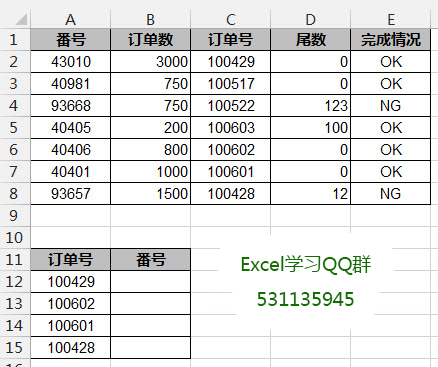# excel中lookup函数经典案例：逆向查找番号

[日期：2014-07-30]   来源：IT部落窝  作者：IT部落窝   阅读：2720[字体： ]

excel中lookup函数是非常常用的查找函数。本文我们一起来认识excel中lookup函数的经典查找模式：

Lookup(1,0/((条件1)*(条件2)*(条件n)),返回值)

excel中lookup函数案例一：根据订单号，逆向查找番号我们只需要套上文章最开始的excel中lookup函数查找模式即可：
=LOOKUP(1,0/(\$C\$2:\$C\$8=A12),\$A\$2:\$A\$8)

0/(\$C\$2:\$C\$8=A12)的作用就是将符合条件的值转换成0，其他转换成错误值。在这里认识下有独孤九剑之称的【F9】键。

=LOOKUP(1,0/(\$C\$2:\$C\$8=A12),\$A\$2:\$A\$8)

=LOOKUP(1,0/{TRUE;FALSE;FALSE;FALSE;FALSE;FALSE;FALSE},\$A\$2:\$A\$8)

=LOOKUP(1,0/(\$C\$2:\$C\$8=A12),\$A\$2:\$A\$8)

=LOOKUP(1,{0;#DIV/0!;#DIV/0!;#DIV/0!;#DIV/0!;#DIV/0!;#DIV/0!},\$A\$2:\$A\$8)
Lookup喜欢以大欺小，用1找0，就是返回最后一个0的对应值。前提是有多个对应值，没有的话就直接返回0的对应值。

excel中lookup函数案例二：根据俗称跟订单号，查找订单数=LOOKUP(1,0/((\$A\$2:\$A\$10=A14)*(\$B\$2:\$B\$10=B14)),\$C\$2:\$C\$10)

如果需要学习更多的excel知识，请用手机微信扫一扫关注微信公众号。分享到： QQ空间 新浪微博 腾讯微博 人人网